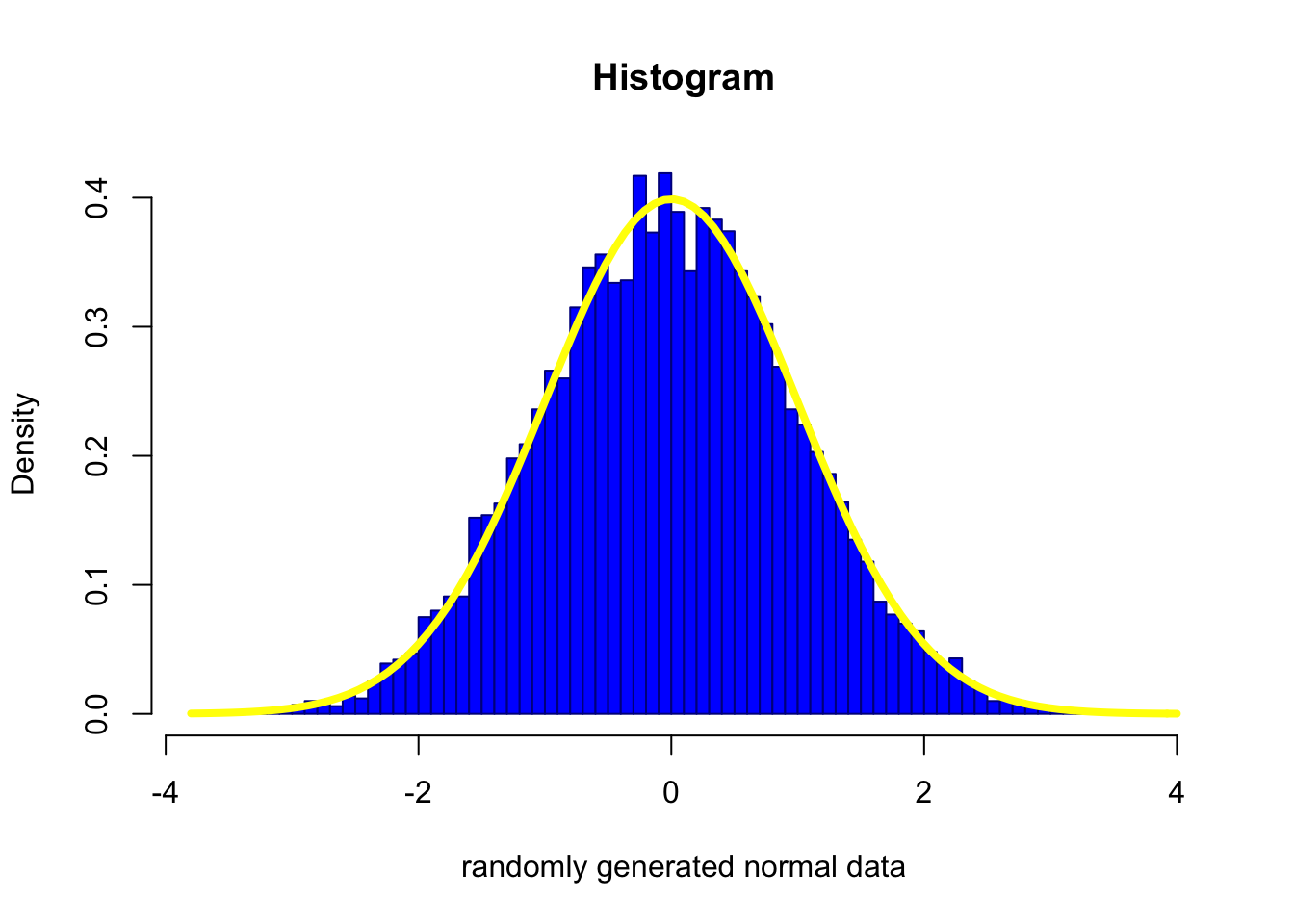Your verification ID is: guDlT7MCuIOFFHSbB3jPFN5QLaQ Big Computing: An Example of Plotting a Histogram in base R

## Doing plots in R is easy, and there are countless types of graphs you can do in base R, Lattice and other packages like ggplot2. One of the most used plot is a simple Histogram. Histogram ared use to plot the number of times a element in a column has a certain value. It is a great way to first visualise a single column of data. In order to use the histogram plot the values must be numeric.

Lets do a simple plot of data
``````numbers<-c(1,2,2,3,3,3,4,4,4,4,5,5,5,5,5,6,6,6,6,6,6)
hist(numbers)``````Well, that is a little funky. The histgrom is technically correct, but what has happened here is the program determined the breaks which resulted in the integers 1 and 2 being put together in the same bin. The way to fix this is to add the break term to the Histgram Plot.
``hist(numbers,breaks=c(0,1,2,3,4,5,6))``The issue here is you really need to know what you have. A better method may be to create a lot of bins (here I use the length of the numbers vector).
``hist(numbers,breaks=length(numbers))``Typically the historgram is used to determine if the data you have visually matches a certian distribution like normal, t, poisson, gamma, etc. In order to do that we need to add another element to the histogram plot and add a plot of the actual curve of the distrbution to our histogram. In the call for the histogram we need to add the term freq=FALSE. This will convert the frequencies of the Histogram from pure counts to ratios that add up to 1. Lets see what this looks like with a sample of 10,000 random normal values
``````dist=rnorm(10000)
hist(dist,freq=FALSE)``````hist(dist,breaks=100,freq=FALSE,col="blue",border="dark blue",main="Histogram",xlab="randomly generated normal data")